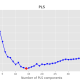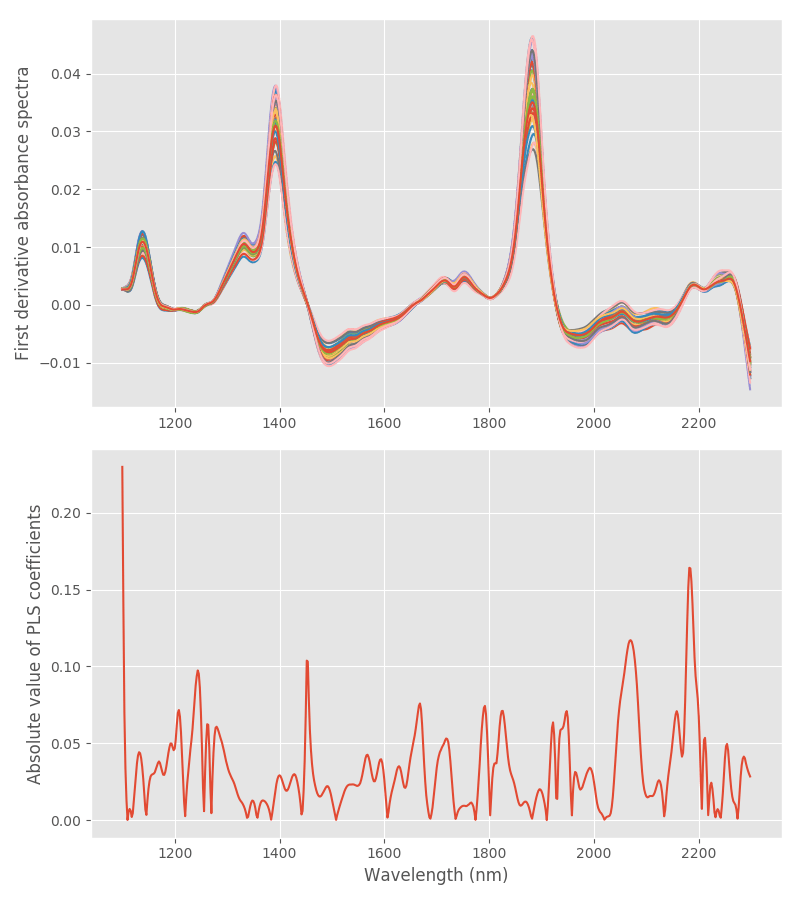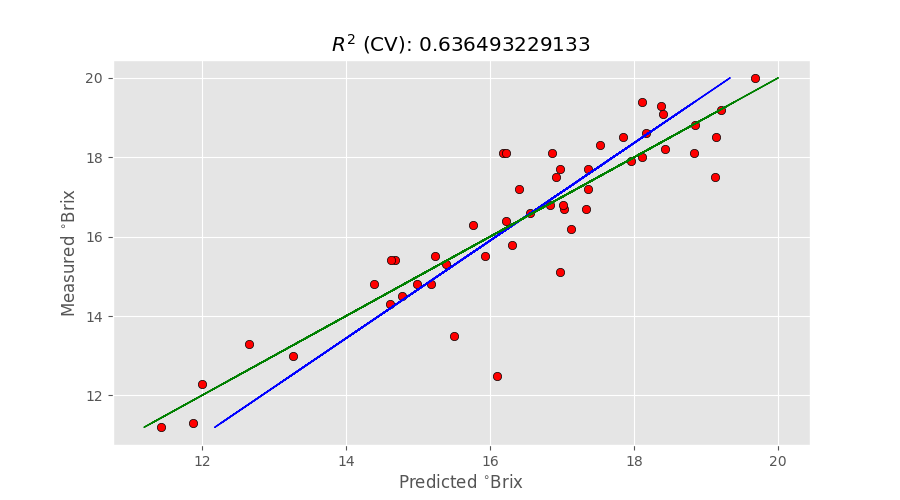###### Partial Least Squares Regression in Python
06/14/2018###### Two scatter correction techniques for NIR spectroscopy in Python
07/21/2018Welcome to our new technical tutorial on Python chemometrics; today we will be discussing a variable selection method for PLS in Python.

In other posts we’ve covered Principal Component Regression (PCR) and the basics of Partial Least Squares (PLS) regression. At the heart of all regression methods is the idea of correlating measured spectra of a substance to be analysed, with known reference values of the same substance. In this way one can build a calibration model that ‘translates’ the spectrum into the value of interest. A robust calibration will then enable to obtain the value (or values) of interest straight form the spectra.

PCR is quite simply a regression model built using a number of principal components derived using PCA. In our last post on PCR, we discussed how PCR is nice and simple, but limited by the fact that it does not take into account anything other than the regression data. That is, our primary reference data are not considered when building a PCR model. That is obviously not optimal, and PLS is a way to fix that.

The quality of the calibration is usually measured according to different metrics, and you can take a look at our previous posts (and countless other online resources) to get an idea of these metrics.

In this post we will discuss what variable selection is, and how it can be used to improve the fidelity of the calibration. Hope you’ll enjoy it.

# Variable selection in chemometrics

The idea behind variable selection in chemometrics is that when it comes to spectral measurements not all wavelengths are created equals. In visible and NIR spectroscopy especially, it is often hard to predict in advance which wavelength bands will contain most of the signal related to the analyte we want to measure. So, as a first shot, we measure the whole range allowed by our instrument, and then figure out later which bands are more relevant for our calibration.

To say the same thing in a bit more quantitative way, we want to check which wavelength bands lead to a better quality model. Actually, in practice, we check which bands give a worse quality model, so we can get rid of them.

This seems logical enough, but I’ve deliberately left a fundamental piece of the puzzle out. How do we even start breaking our spectrum into bands and searching for the worst performing ones?

For this example we’ll take a simple approach: we’ll filter out wavelength bands based on the strength of the related regression coefficients. Let’s understand what that means.

# Feature selection by filtering

Feature selection by filtering is one of the simplest ways of performing wavelength selection. For an overview of the methods that are currently used, check out this excellent review paper by T. Mehmood et al. (available for download here).

The idea behind this method is very simple, and can be summarised in the following:

1. Optimise the PLS regression using the full spectrum, for instance using cross-validation or prediction data to quantify its quality.
2. Extract the regression coefficients form the best model. Each regression coefficient uniquely associates each wavelength with the response. A low absolute value of the regression coefficient means that specific wavelength has a low correlation with the quantity of interest.
3. Discard the lowest correlation wavelengths according to some rule. These wavelengths are the ones that typically worsen the quality of the calibration model, therefore by discarding them effectively we expect to improve the metrics associated with our prediction or cross-validation.

One option to discard the lowest correlation wavelengths is to set a threshold and get rid of all wavelengths whose regression coefficients (in absolute value) fall below that threshold. However this method is very sensitive to the choice of threshold, which tends to be a subjective choice, or requires a trial-and-error approach.

Another approach, that is much less subjective, is to discard one wavelength at a time (the one with the lowest absolute value of the associated regression coefficient) and rebuild the calibration model. By choosing the MSE (means square error) of prediction or cross-validation as metric, the procedure is iterated until the MSE decreases. At some point, removing wavelengths will produce a worse calibration, and that is the stopping criterion for the iteration.

Alternatively, one could simply remove a fixed number of wavelengths iteratively, and then check for which number of removed wavelengths the MSE is minimised. Either way we have a method that does not depend on the subjective choice of the threshold and can be applied without changes regardless of the data set being analysed.

One caveat of this method, at least in its simple implementation is that it may get a bit slow for large datasets.

# Extracting PLS regression coefficients: Python code

We start, as usual, with the list of import you’ll need to run this example

Next, we import the data, calibration and calculate the derivatives.

Our aim for this tutorial is to define a function that will simultaneously optimise the number of PLS components and the sequence of wavelengths to minimise the MSE in cross-validation (take a look at our previous post on PLS regression if you want to brush up on the basic PLS code and the terminology). Before doing that however, let’s look at the basic feature-selection idea: the absolute value of the PLS coefficients.

What we have done is a basic PLS regression with 8 components and used it to fir the first derivative data. The PLS coefficients of this fit can be accessed by `pls.coef_[:,0]`, that is the first (and in this case only) column of the `pls.coef_` object. It is worth reiterating that these are the regression coefficients that quantify the strength of the association between each wavelength and the response. We are interested only in the absolute value of these coefficients, as large positive and large negative coefficients are equally important for our regression, denoting large positive or large negative correlation with the response respectively. In other words we want to identify and discard the regression coefficients that are close to zero: they are the ones with poor correlation with the response.

The association between each coefficient and the spectral feature can be visualised using the the second part of the code above, producing this plot.As you can see, a number of wavelengths are associated with fairly small regression coefficients. We want to use this basic idea to identify and discard small coefficients iteratively, starting from the smallest, and optimise the PLS regression at the same time.

# NIR variable selection for PLS regression

We are almost ready to write down the code for the complete function we want to use. One last thing to point out is the trick we’ll use to discard small PLS regression coefficients.

What we have done is to extract the sequence of indices corresponding to sorting the absolute value of the PLS regression coefficients in ascending order, and use that sequence to sort the spectra accordingly. Note that shuffling around the spectral component has no influence whatsoever on the PLS regression, but it enables us to easily discard one component at a time.

OK, we are now ready to reveal the entire optimisation function.

This function works by running a PLS regression with a given number of components (up to a specified maximum), then filtering out one regression coefficient at a time up to the maximum number allowed. All data are stored in the 2D array mse. At the end of the double loop we search for the global minimum of mse excluding the zeros. That will give us the number of components and the wavelength bands that corresponds to the optimal cross-validation MSE.

To give you an idea of the huge improvement we can get with this approach, let’s compare the result obtained using the full spectrum with the one optimised by variable selection. Using the full spectrum (and the code of the previous post, modified to calculate cross-validation) we find that the optimal number of PLS components is 8 (The code is reproduced at the end of this post). This is the output

`R2 calib: 0.778 R2 CV: 0.438 MSE calib: 1.035 MSE CV: 2.618`And this is the results after running the optimisation.
` Optimised number of PLS components: 9 Wavelengths to be discarded 452 Optimised MSE 1.74293961079`

R2 calib: 0.813
R2 CV: 0.636
MSE calib: 0.873
MSE CV: 1.694The R2 score jumped from 0.44 to 0.64, while the MSE cross-validation decreased from 2.6 to 1.7! That amounted to discarding 452 wavelengths out of 600, that is finding the truly significant bands for Brix calibration.

As a sanity check, we can plot the discarded bands on top of the absorbance spectra. The code is as follows.

This is the plot of the first derivative spectra with overlapped the discarded bands in red. Note how the main water peaks are actually discarded.Thanks for reading our tutorial describing a variable selection method for PLS in Python. I hope you enjoyed it and until next time!

PS Here’s the code for a single instance of PLS modified to work with cross-validation.##### Daniel Pelliccia
Physicist and an entrepreneur. Founder and Managing Director at Instruments & Data Tools, specialising in optical design and analytical instrumentation. Founder at Rubens Technologies, the intelligence system for the fresh fruit export industry.